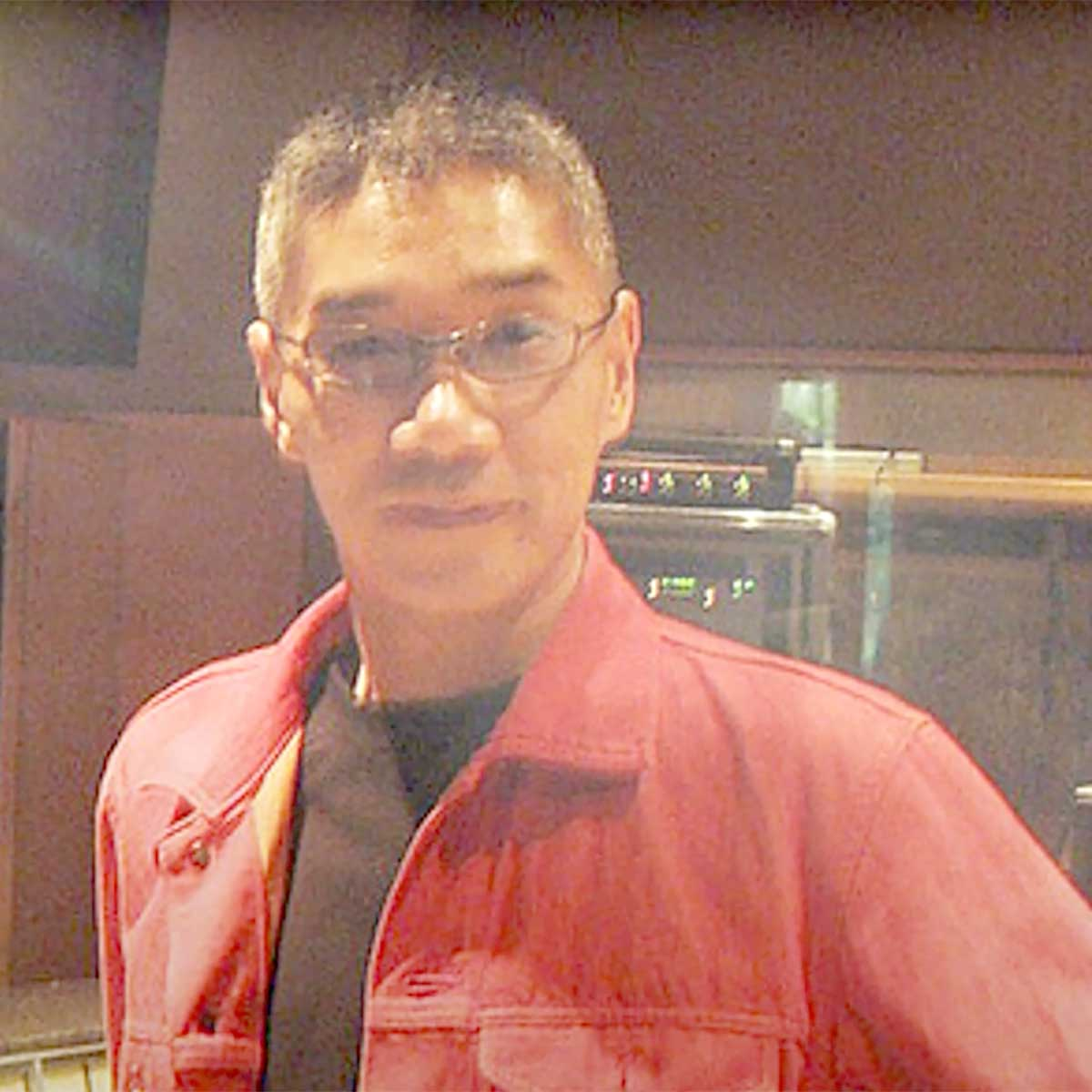# Kenji Yamamoto

1 Music Sheets

• ###### Birth Name:

Kenji Yamamoto

• ###### Born:

1st July 1958

• ###### Genres:

Kenji Yamamoto (山本 健司, Yamamoto Kenji, born July 1, 1958) is a Japanese music composer and arranger who has been responsible for producing and composing soundtracks, including opening and ending sequence themes for various anime, tokusatsu and video game projects in the 1980s, 1990s, and 2000s. Most notably, he has worked on soundtracks of various Dragon Ball video games.

Credit: Wikipedia

## Artist's Music Sheets

•[gcJ] s P i g s P i [gZJ] s P i g s P i [zhg] s P i g s P i [lhg] s P i g s P i [gcJ] s P i g s P i [gZJ] s P i g s P i [zhg] s P i g s P i [lhg] s P i g s P i q E t i| |[qZ] [zE] [tZ] [ic]| |[qZ] [zE] [tZ] [vi]| |[zq] [lE] t i| t E| [qZ] [zE] [tZ] [ic]||J| [qZ] [zE] [tZ] [vi]||h| [qZ] [zE] [tZ] [ic]| |[zq] [JE] t i| t E| [qc] E t [iZ]||z| [ql] E t [zi]||J| [ql] E t i| |q E t i| t E| [qc] E t [iZ]||z| [ql] E t [zi]||Z| [zq] E t i| |q E t i| t E| [qZ] [zE] [tZ] [ic]| |[qZ] [zE] [tZ] [ic]| |[qZ] [zE] [tZ] [ic]| z| J [ql] E t i| t E| [qZ] [zE] [tZ] [ic]| |[qZ] [zE] [tZ] [ic]| |[qZ] [zE] [tZ] [ic]| z| J [ql] E t i| t E| q E t i| |[ql]z[ZE]c[vt]b[iB]mzZcvcZzm[qc]Z[zE]m[tc]Z[zi]mcZzmBVvm[qB]V[vE]c[tZ]z[li]JcZzlJHhg[qJ]H[hE]g[tD]d[ih]gDdsPOoi q E t i| |[slh] q [slhE] t [slih]| [slh]| [slh] q [slhE] t [slih]| [slh]| [slh] q E t i| |[ql]z[ZE]c[vt]b[iB]mzZcvcZzm[qc]Z[zE]m[tc]Z[zi]mcZzmBVvm[qB]V[vE]c[tZ]z[li]JcZzlJHhg[qJ]H[hE]g[tD]d[ih]gDdsPOoi q E t i| |[slh] q [slhE] t [slih]| [slh]| [slh] q [slhE] t [slih]| [slh]| [slh] q E t i| |[wtD8] d D [wtg8]||[wt8]| [q^ED] d D [qh^E]||[q^E]| [WD(%] d D [gW(%]||[W(%]| [qd^E] P| [q^E]| 4 ^| [wtD8] d D [wtg8]||[wt8]| [q^ED] d D [qh^E]||[q^E]| [WD(%] d D [gW(%]||[W(%]| [qd^E] P| [q^E]| 4 ^| [sig1] 5 w [tYD]||[ywd]| [ts^] 4 q [ydE]||[qPE]| [YD%] [yd@] [YD(] [igW]||(| [yd^] [PE4] q E||q| [sig1] 5 w [tYD]||[ywd]| [ts^] 4 q [ydE]||[qPE]| [YD%] [yd@] [YD(] [igW]||(| [yd^] [PE4] q E||q| [tog]||D||d| [siE]||d||P| [sYW]| |[iE]| |[tog]||D||d| [siE]||d||D| [hYW] d| |[iE]| |[shZD] z J l| |[shD]| |[vodP]| |v c B [odZP]| |[zpid] Z c v| |[pid]| |[zsoD] Z J l| |[soD]| |[ts8] g h l s g h l s g h l s g h l [s^E] g h l s g h l s g h l s g h l [sW%] g h l s g h l s g h l s g h l [s^E] g h l s g h l s g h l s g h l [s81] g h l s g h l s g h l s g h l [s^] g h l s g h l s g h l s g h l [s%] g h l s g h l s g h l s g h l [sq4] g h l s g h l [s@(] g h l [s92] g h l [s81] g h l s g h l s g h l s g h l

Level: 6
Length: 03:44
Intermediate